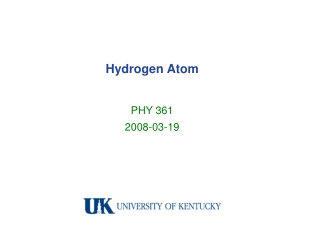DownloadDownload PresentationHydrogen Atom

# Hydrogen Atom

Download Presentation## Hydrogen Atom

- - - - - - - - - - - - - - - - - - - - - - - - - - - E N D - - - - - - - - - - - - - - - - - - - - - - - - - - -
##### Presentation Transcript

1. Hydrogen Atom PHY 361 2008-03-19

2. review of Lz operator, eigenfunction, eigenvalues rotational kinetic energy traveling and standing waves spherical coordinates definition Laplacian operator Schrödinger’s equation in spherical coordinates separation of angular variables: L2 and Lz differential equations spherical harmonics and eigenvalues vector model of quantum angular momentum radial wavefunctions effective radial potential – centrifugal `force’ radial wave functions hydrogenic orbitals Outline

3. Spherical Coordinates

4. Cylindrical vs. Spherical Coordinates Laplacian: Schrödinger Equation: Lz2 / 2I L2 / 2I

5. Spherical Harmonics L2 Ylm=l(l+1)Ylm s 1 Lz Ylm= m Ylm x, y p z x, y x2+y2, xy xz, yz d 3z2-1 xz, yz x2+y2, xy f …

6. Vector model of quantized angular momentum l = 0, 1, 2, … m = -1, -l+1, … l-1, l

7. Radial equation – effective potential

8. Radial hydrogenic wavefunctions

9. Hydrogenic orbitals http://www.orbitals.com/orb/